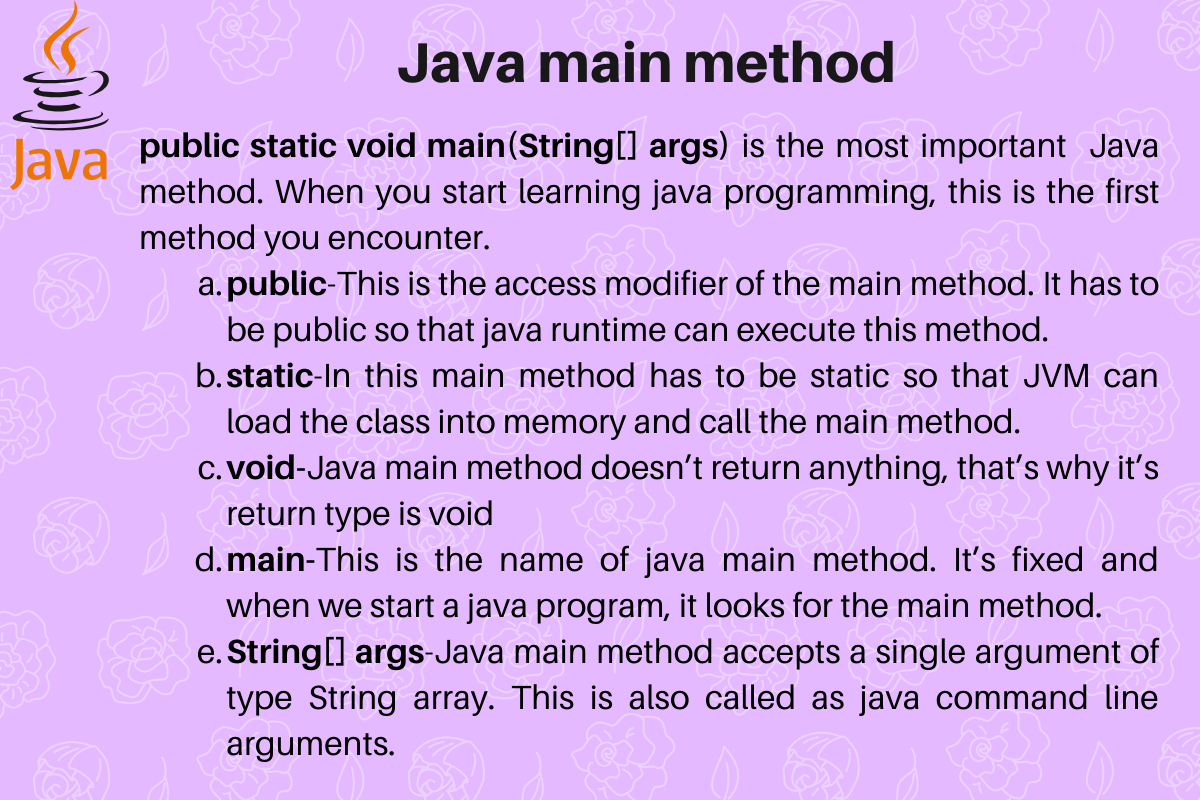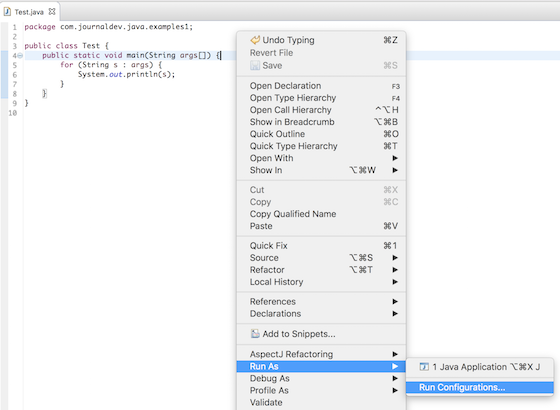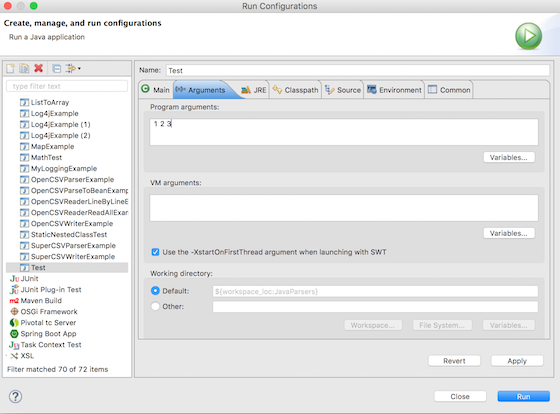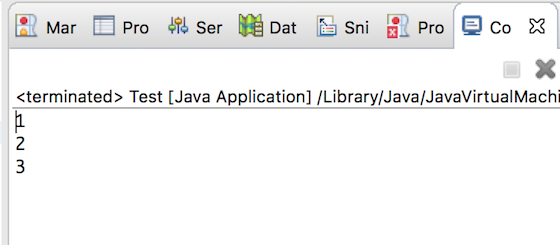# public static void main(String[] args) - Java main method

Published on August 3, 2022By Pankaj
Developer and author at DigitalOcean.While we believe that this content benefits our community, we have not yet thoroughly reviewed it. If you have any suggestions for improvements, please let us know by clicking the “report an issue“ button at the bottom of the tutorial.

`public static void main(String[] args)` is the most important Java method. When you start learning java programming, this is the first method you encounter. Remember the first Java Hello World program you wrote that runs and prints “Hello World”?

## public static void main(String[] args)

Java main method is the entry point of any java program. Its syntax is always `public static void main(String[] args)`. You can only change the name of String array argument, for example you can change `args` to `myStringArgs`. Also String array argument can be written as `String... args` or `String args[]`.Let’s look at the java main method closely and try to understand each of its parts.

### public

This is the access modifier of the main method. It has to be `public` so that java runtime can execute this method. Remember that if you make any method non-public then it’s not allowed to be executed by any program, there are some access restrictions applied. So it means that the main method has to be public. Let’s see what happens if we define the main method as non-public.

``````public class Test {

static void main(String[] args){

System.out.println("Hello World");

}
}
``````
``````\$ javac Test.java
\$ java Test
public static void main(String[] args)
or a JavaFX application class must extend javafx.application.Application
\$
``````

### static

When java runtime starts, there is no object of the class present. That’s why the main method has to be static so that JVM can load the class into memory and call the main method. If the main method won’t be static, JVM would not be able to call it because there is no object of the class is present. Let’s see what happens when we remove static from java main method.

``````public class Test {

public void main(String[] args){

System.out.println("Hello World");

}
}
``````
``````\$ javac Test.java
\$ java Test
Error: Main method is not static in class Test, please define the main method as:
public static void main(String[] args)
\$
``````

### void

Java programming mandates that every method provide the return type. Java main method doesn’t return anything, that’s why it’s return type is `void`. This has been done to keep things simple because once the main method is finished executing, java program terminates. So there is no point in returning anything, there is nothing that can be done for the returned object by JVM. If we try to return something from the main method, it will give compilation error as an unexpected return value. For example, if we have the main method like below.

``````public class Test {

public static void main(String[] args){

return 0;
}
}
``````

We get below error when above program is compiled.

``````\$ javac Test.java
Test.java:5: error: incompatible types: unexpected return value
return 0;
^
1 error
\$
``````

### main

This is the name of java main method. It’s fixed and when we start a java program, it looks for the main method. For example, if we have a class like below.

``````public class Test {

public static void mymain(String[] args){

System.out.println("Hello World");
}
}
``````

And we try to run this program, it will throw an error that the main method is not found.

``````\$ javac Test.java
\$ java Test
public static void main(String[] args)
or a JavaFX application class must extend javafx.application.Application
\$
``````

### String[] args

Java main method accepts a single argument of type String array. This is also called as java command line arguments. Let’s have a look at the example of using java command line arguments.

``````public class Test {

public static void main(String[] args){

for(String s : args){
System.out.println(s);
}

}
}
``````

Above is a simple program where we are printing the command line arguments. Let’s see how to pass command line arguments when executing above program.

``````\$ javac Test.java
\$ java Test 1 2 3
1
2
3
\$ java Test "Hello World" "Pankaj Kumar"
Hello World
Pankaj Kumar
\$ java Test
\$
``````

### Java main method command line arguments in Eclipse

Below images show how to pass command line arguments when you are executing a java program in Eclipse.That’s all for `public static void main(String[] args)`, java main method.Pankaj

author

Developer and author at DigitalOcean.

#### Still looking for an answer?

import java.util.Scanner; public class Main { public static void main (String[] args){ Scanner input= new Scanner(System.in); boolean isOnRepeat=true; while(isOnRepeat){ System.out.println (“play the currentsong”); System.out.println(“do you want to change the song, If so answer yes”); String userInput = input.next(); if(userInput.equals(“yes”)) { isOnRepeat=false; } } System.out.println (“play the next song”); } }

- Jeyaharini

public class Main { public static void main(String[] args) { int a[]=new int[]{12,2,6,7,11}; int b[]=new int[]{2,6,7,11}; int i=0,j; int way=0; int f; int c[]=new int; for(i=1;i<=12;i++) { f=0; for(j=0;j<4;j++) { if(i==a[j]) f=1; } if(f==0) c[i-1]=i; else c[i-1]=0; if(i%2==0) { if(c[i-1]!=0 && c[i-2]!=0) way++; } } for(i=0;i<10;i++) { if(c[i]!=0 && c[i+2]!=0) way++; } System.out.println(“”+way); } }

- RK

Arrays in java are all supposed to have a fixed size, but the array of strings that’s passed to main (String[] args) doesn’t. Is this a hard-coded exception to the rule? Are there any other such examples?

- Jackson

- Ackeem

Help me determine the output for the given code snippet: public class App { public static void main( String[] args ) { for(;;) { System.out.println(“Hi”); } } }

- Dia

please help me find the errors in this code import java.util.Scanner;    public class StringLength  {    public void main(String[] args)    {      Scanner scan = new Scanner(System);          String message = "Enter a sentence: ";      boolean valid = false;      String input = “”;      int indexOfFullStop = 0;      int indexOfQuestion = 0;      int indexOfExclamation = 0;      int lastIndex = 0;          do      (        System.out.print(message);     input = scan.nextLine();             indexOfFullStop = input.indexOf(‘.’);        indexOfQuestion = input.indexOf(‘?’);        indexOfExclamation = input.indexOf(‘!’);           if((indexOfFullStop != 0) && (indexOfQuestion != 0) && (indexOfExclamation != 0))        {          //the sentence ended correctly          valid = true;                //get the index of the final character that ends the senten ce          if(indexOfFu llStop != ‐1)          {            lastIndex = indexOfFullStop;          }          else          {            if(indexOfQuestion == ‐1)                     lastIndex = indexOfQuestion;            }            else            {              lastIndex = indexOfExclamation;            }          }        }        else        {        //the sentence did not end with one of the correct characters          message = “A sentence must end with a . OR ! OR ?” +                 "\nEnter a sentence again: ";        }           }while(valid)          int length = lastIndex;          message = “The length of the sentence is " + length + " characters”;      System.out.println(message);        }  }

- nhlanhla

class Test { public static void main (String args[]) { String s = “Hello Java” ; for(int i=0; 0 ; i++) { System.out.println( s ); break; } } } Explain why the above code give the compile-time error? Correct the above code.

- kim ka

My teacher writes as public static void main(String St[]) and it is executed. My question is that (1) what are the maximum possibilities to write in different string names? (2) Why we need to use array in different names? (3) What is the purpose of using different name for String?

- Fareha

public class Main { private String isbn, author, title, publisher, publishdate; private int price; private String borrower,category; private int payment; private int numberofdays; public Main(){ isbn=“unassigned”; author=“unnasigned”; title=“unnasigned”; publisher=“unnasigned”; publishdate=“unnasigned”; price= 0; } public void setNumberofdays(int nd){ numberofdays = nd; } public void setBorrower(String b){ category = b; } public void setCategory (String c){ category= c; } public void setBookTitle(String t){ title = t; } public void setPublisher(String p){ publisher = p; } public void setAuthor(String a){ author = a; } public void setPublishDate(String pd){ publishdate = pd; } public void setpayment(int pm){ payment = pm; } public void setISBN(String i){ isbn = i; } public void setPrice(int p){ price = p; } public void setPayment (int nd){ payment = nd*5; } public int getNumberofdays(){ return numberofdays; } public String getAuthor(){ return author; } public String getISBN(){ return isbn; } public String getBorrower(){ return borrower; } public String getCategory(){ return category; } public String getBookTitle(){ return title; } public int getpayment(){ return payment; } public String getPublisher(){ return publisher; } public String getPublishDate(){ return publishdate; } public int getPrice(){ return price; } public int getPayment(){ return payment; } } Why my code is not working??

- Ricardo

are these correct? public static main(String[ ] args) public static void[ ] main(String[ ] args) public int static main(String[ ] args) public static void mian (String args)

- yon